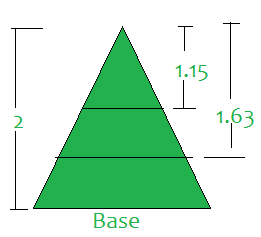Split given isosceles triangle of height H into N equal parts

• Difficulty Level : Easy
• Last Updated : 18 Jun, 2021

Given an integer N and an isosceles triangle consisting of height H, the task is to find (N – 1) points on the triangle such that the line passing through these points and parallel to the base of the triangle, divide the total area into N equal parts.

Examples:

Input: N = 3, H = 2
Output: 1.15 1.63
Explanation: Make cuts at point 1.15 and 1.63 as shown below:Input: N = 2, H = 1000
Output: 70710.67

Approach: The problem can be solved by observing the following properties:

Divide the triangle such that (xi / h)2 = i / N
=> xi = h*√(i/n)
xi = height of ith cut from the top vertex of the triangle

Follow the steps below to solve the problem:

1. Iterate over the range [1, N – 1].
2. In every ith iteration, print the value of xi using the above formula.

Below is the implementation of the above approach:

C++

 // C++ Code for above approach#include using namespace std; // Function to divide the isosceles triangle// in equal parts by making N-1 cuts// parallel to the basevoid findPoint(int n, int h){       // Iterate over the range [1, n - 1]    for (int i = 1; i < n; i++)        printf("%.2f ", sqrt(i / (n*1.0)) * h);} // Driver codeint main(){  // Given N  int n = 3;   // Given H  int h = 2;   // Function call  findPoint(n, h);   return 0;} // This code is contributed by mohit kumar 29

Java

 // Java Code for above approachimport java.util.*;class GFG{     // Function to divide the isosceles triangle    // in equal parts by making N-1 cuts    // parallel to the base    static void findPoint(int n, int h)    {         // Iterate over the range [1, n - 1]        for (int i = 1; i < n; i++)            System.out.printf("%.2f ",                    Math.sqrt(i / (n * 1.0)) * h);    }     // Driver code    public static void main(String[] args)    {               // Given N        int n = 3;         // Given H        int h = 2;         // Function call        findPoint(n, h);    }} // This code is contributed by shikhasingrajput

Python3

 # Python Code for above approach  # Function to divide the isosceles triangle# in equal parts by making N-1 cuts# parallel to the basedef findPoint(n, h):      # Iterate over the range [1, n - 1]    for i in range(1, n):        print("{0:.2f}".format(((i / n) ** 0.5) * h), end =' ')  # Driver Codeif __name__ == '__main__':     # Given N    n = 3     # Given H    h = 2     # Function call    findPoint(n, h)

C#

 // C# Code for above approachusing System;class GFG{   // Function to divide the isosceles triangle  // in equal parts by making N-1 cuts  // parallel to the base  static void findPoint(int n, int h)  {     // Iterate over the range [1, n - 1]    for (int i = 1; i < n; i++)      Console.Write("{0:F2} ",                    Math.Sqrt(i / (n * 1.0)) * h);  }   // Driver code  public static void Main(String[] args)  {     // Given N    int n = 3;     // Given H    int h = 2;     // Function call    findPoint(n, h);  }} // This code is contributed by shikhasingrajput

Javascript


Output:
1.15 1.63

Time Complexity: O(N)
Auxiliary Space: O(1)

My Personal Notes arrow_drop_up